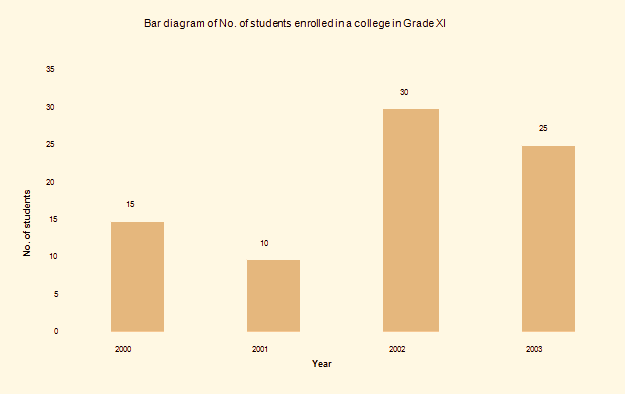# Simple Bar Diagram - Economics Notes Grade XI

### Commerce Notes

☰   Related Articles

#### Scarcity And Choice

Concepts of Scarcity And Choice

#### Introduction to Statistics# Simple Bar Diagram

Simple Bar Diagram
Simple bar diagrams are used when there is only one characteristic of the data and there may be many bars as the number of observations of the same variable. For example, the data obtained on the number of successful students in a college in various years can be presented by simple diagrams. It consists of a set of equidistant rectangles of equal width which heights represent the value of the variable.

Example:
Represent the following data of a number of students enrolled in a college in grade XI.

 No. of Students Year 2000 15 2001 10 2002 30 2003 25Above diagram is the example of Simple Bar Diagram.

## You may also like to read:

Join with us on social media to see our updates on your feed.